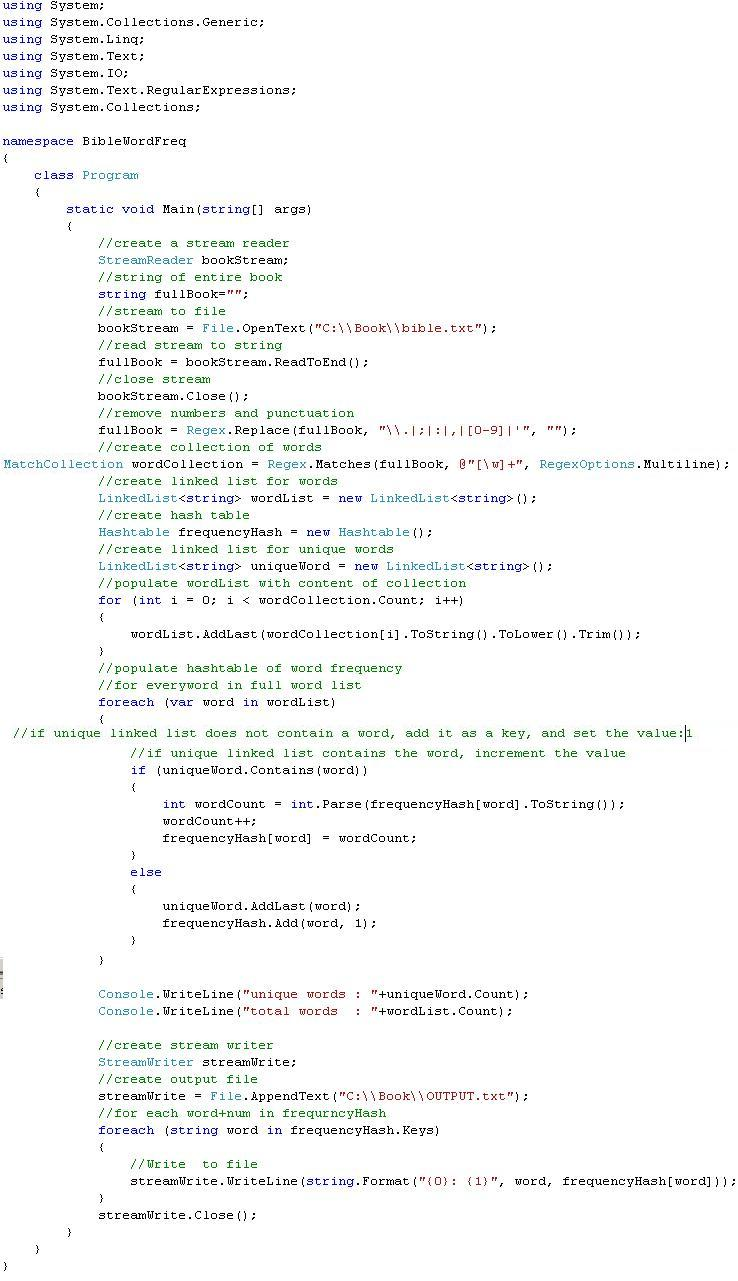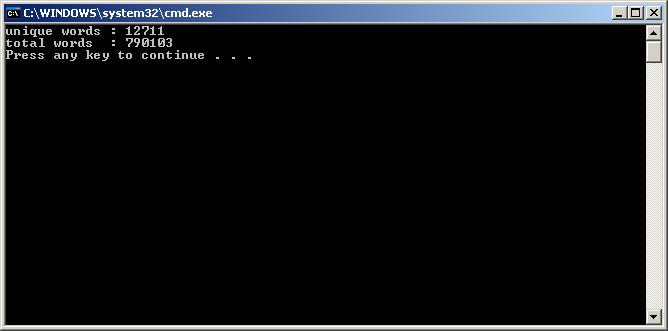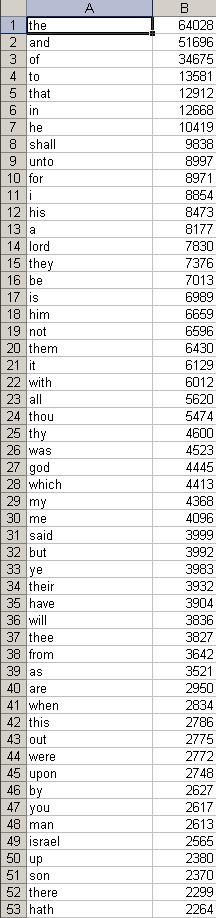# C#: How to parse every word in a book and output the frequency of repeating words: The Holy Bible

The book being parsed in this example is the King James Holy Bible bible.txtColumn A is the word, Column B is the frequencymicrosoft excel format :  bibFreqxls.xls

xml format                     :  bibFreqxml.txt

[csharp]
using System;
using System.Collections.Generic;
using System.Linq;
using System.Text;
using System.IO;
using System.Text.RegularExpressions;
using System.Collections;

namespace BibleWordFreq
{
class Program
{
static void Main(string[] args)
{
//string of entire book
string fullBook=””;
//stream to file
bookStream = File.OpenText(“C:\\Book\\bible.txt”);
//close stream
bookStream.Close();
//remove numbers and punctuation
fullBook = Regex.Replace(fullBook, “\\.|;|:|,|[0-9]|'”, “”);
//create collection of words
MatchCollection wordCollection = Regex.Matches(fullBook, @”[\w]+”, RegexOptions.Multiline);
//create hash table
Hashtable frequencyHash = new Hashtable();
//create linked list for unique words
//populate wordList with content of collection
for (int i = 0; i < wordCollection.Count; i++)
{
}
//populate hashtable of word frequency
//for everyword in full word list
foreach (var word in wordList)
{
//if unique linked list does not contain a word, add it as a key, and set the value:1
//if unique linked list contains the word, increment the value
if (uniqueWord.Contains(word))
{
int wordCount = int.Parse(frequencyHash[word].ToString());
wordCount++;
frequencyHash[word] = wordCount;
}
else
{
}
}

Console.WriteLine(“unique words : “+uniqueWord.Count);
Console.WriteLine(“total words  : “+wordList.Count);

//create stream writer
StreamWriter streamWrite;
//create output file
streamWrite = File.AppendText(“C:\\Book\\OUTPUT.txt”);
//for each word+num in frequrncyHash
foreach (string word in frequencyHash.Keys)
{
//Write  to file
streamWrite.WriteLine(string.Format(“{0}: {1}”, word, frequencyHash[word]));
}
streamWrite.Close();
}
}
}

[/csharp]

## 6 thoughts on “C#: How to parse every word in a book and output the frequency of repeating words: The Holy Bible”

1. Venkat B

This is very helpful coding. Can we optimize it using dictionary instead of linked list? Also I need to convert where ever non alphanumeric is there it should be treated as seperator. Can you suggest?

The following dictionary implementation is about 10 times faster:

``` using System; using System.IO; using System.Collections.Generic; using System.Text.RegularExpressions;```

``` namespace BibleWordFreq { class Program { static void Main(string[] args) { string fullBook = File.ReadAllText("C:\\Book\\bible.txt").ToLower(); //remove numbers and punctuation fullBook = Regex.Replace(fullBook, "\\.|;|:|,|[0-9]|’", ""); //create collection of words var wordCollection = Regex.Matches(fullBook, @"[\w]+"); //calculate word frequencies var dict = new Dictionary(); for (int i = 0; i < wordCollection.Count; i++) { string word = wordCollection[i].Value; if (!dict.ContainsKey(word)) dict[word] = 1; else ++dict[word]; } Console.WriteLine("unique words : " + dict.Count); Console.WriteLine("total words : " + wordCollection.Count); ```

``` using (StreamWriter streamWrite = new StreamWriter("C:\\Book\\OUTPUT.txt")) { foreach (KeyValuePair kv in dict) streamWrite.WriteLine("{0}: {1}", kv.Key, kv.Value); } } } } ```